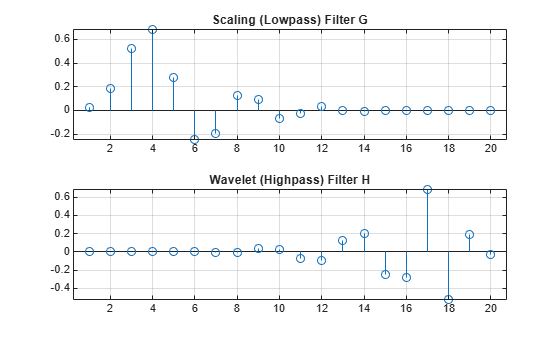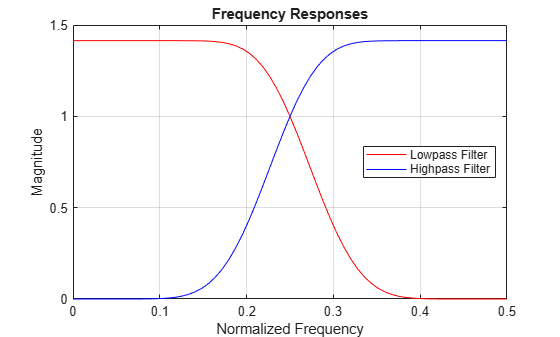# qmf

Scaling and Wavelet Filter

## Syntax

```Y = qmf(X,P) Y = qmf(X) Y = qmf(X,0) ```

## Description

`Y = qmf(X,P)` changes the signs of the even index elements of the reversed vector filter coefficients `X` if `P` is `0`. If `P` is `1`, the signs of the odd index elements are reversed. Changing `P` changes the phase of the Fourier transform of the resulting wavelet filter by π radians.

`Y = qmf(X)` is equivalent to `Y = qmf(X,0)`.

Let `x` be a finite energy signal. Two filters F0 and F1 are quadrature mirror filters (QMF) if, for any x,

`${‖{y}_{0}‖}^{2}+{‖{y}_{1}‖}^{2}={‖x‖}^{2}$`

where y0 is a decimated version of the signal x filtered with F0 so y0 defined by x0 = F0(x) and y0(n) = x0(2n), and similarly, y1 is defined by x1 = F1(x) and y1(n) = x1(2n). This property ensures a perfect reconstruction of the associated two-channel filter banks scheme (see Strang-Nguyen p. 103).

For example, if F0 is a Daubechies scaling filter with norm equal to 1 and F1 = `qmf`(F0), then the transfer functions F0(z) and F1(z) of the filters F0 and F1 satisfy the condition (see the example for `db10`):

`$|{F}_{0}\left(z\right){|}^{2}+|{F}_{1}\left(z\right){|}^{2}=2.$`

## Examples

collapse all

This example shows how to create a quadrature mirror filter associated with the `db10` wavelet.

Compute the scaling filter associated with the `db10` wavelet.

`sF = dbwavf('db10');`

`dbwavf` normalizes the filter coefficients so that the norm is equal to $1/\sqrt{2}$. Normalize the coefficients so that the filter has norm equal to 1.

`G = sqrt(2)*sF;`

Obtain the wavelet filter coefficients by using `qmf`. Plot the filters.

```H = qmf(G); subplot(2,1,1) stem(G) title('Scaling (Lowpass) Filter G') grid on subplot(2,1,2) stem(H) title('Wavelet (Highpass) Filter H') grid on```Set the DWT extension mode to Periodization. Generate a random signal of length 64. Perform a single-level wavelet decomposition of the signal using `G` and `H`.

`dwtmode('per')`
``` !!!!!!!!!!!!!!!!!!!!!!!!!!!!!!!!!!!!!!!! ! WARNING: Change DWT Extension Mode ! !!!!!!!!!!!!!!!!!!!!!!!!!!!!!!!!!!!!!!!! ***************************************** ** DWT Extension Mode: Periodization ** ***************************************** ```
```n = 64; rng 'default' sig = randn(1,n); [a,d] = dwt(sig,G,H);```

The lengths of the approximation and detail coefficients are both 32. Confirm that the filters preserve energy.

`[sum(sig.^2) sum(a.^2)+sum(d.^2)]`
```ans = 1×2 92.6872 92.6872 ```

Compute the frequency responses of `G` and `H`. Zeropad the filters when taking the Fourier transform.

```n = 128; F = 0:1/n:1-1/n; Gdft = fft(G,n); Hdft = fft(H,n);```

Plot the magnitude of each frequency response.

```figure plot(F(1:n/2+1),abs(Gdft(1:n/2+1)),'r') hold on plot(F(1:n/2+1),abs(Hdft(1:n/2+1)),'b') grid on title('Frequency Responses') xlabel('Normalized Frequency') ylabel('Magnitude') legend('Lowpass Filter','Highpass Filter','Location','east')```Confirm the sum of the squared magnitudes of the frequency responses of `G` and `H` at each frequency is equal to 2.

```sumMagnitudes = abs(Gdft).^2+abs(Hdft).^2; [min(sumMagnitudes) max(sumMagnitudes)]```
```ans = 1×2 2.0000 2.0000 ```

Confirm that the filters are orthonormal.

```df = [G;H]; id = df*df'```
```id = 2×2 1.0000 -0.0000 -0.0000 1.0000 ```

This example shows the effect of setting the phase parameter of the `qmf` function.

Obtain the decomposition low-pass filter associated with a Daubechies wavelet.

`lowfilt = wfilters('db4');`

Use the `qmf` function to obtain the decomposition low-pass filter for a wavelet. Then, compare the signs of the values when the `qmf` phase parameter is set to 0 or 1. The reversed signs indicates a phase shift of $\pi$ radians, which is the same as multiplying the DFT by ${e}^{i\pi }$.

`p0 = qmf(lowfilt,0)`
```p0 = 1×8 0.2304 -0.7148 0.6309 0.0280 -0.1870 -0.0308 0.0329 0.0106 ```
`p1 = qmf(lowfilt,1)`
```p1 = 1×8 -0.2304 0.7148 -0.6309 -0.0280 0.1870 0.0308 -0.0329 -0.0106 ```

Compute the magnitudes and display the difference between them. Unlike the phase, the magnitude is not affected by the sign reversals.

`abs(p0)-abs(p1)`
```ans = 1×8 0 0 0 0 0 0 0 0 ```

## References

Strang, G.; T. Nguyen (1996), Wavelets and Filter Banks, Wellesley-Cambridge Press.

## Extended Capabilities

### C/C++ Code GenerationGenerate C and C++ code using MATLAB® Coder™.

#### Introduced before R2006a

##### Support